OneIdentity

Many users don't understand what the OneIdentiy attribute does.  The cells
below show how this attribute can effect pattern matching.  OneIdentity can
have an effect related to the use of optional arguments and another effect on
functions that also have the Flat attribute.  I was only able to
understanding many of the subtle ways that OneIdentity works after getting
Technical Support from Dave Withoff.

OneIdentity and optional arguments

In the line below we see Default[Power,2] evaluates to (1) and Power has the
attribute OneIdentity.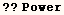Attributes[Power] = {Listable, NumericFunction, OneIdentity, Protected}
 Power/:Default[Power, 2] := 1

Since Default[Power,2] evaluates to (1), the pattern matcher treats (x) as  Power[x,1] when matched with the pattern ( Power[x_,n_.] ).  Using  (n_.)  as  the exponent in power means the exponent is optional, and the Default should  be used when an exponent is not explicitly present.  This causes the  definition in the next cell to work on ( integral[x,x] ).  In addition to  having a value defined for Default[Power,2], the symbol Power has to have the  OneIdentity attribute or the example in the next cell would not work.   Further details of default values are included in the discussion on Default.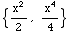In the next output cell we see the rule for integral[x_^(n_.), x_]  isn't
used for integral[x,x]  when Power doesn't have the OneIdentity attribute.To illustrate how Default[expr]  is used, the attribute OneIdentity is
returned to Power, and  the value of Default[Power,2] is changed.  Then the
pattern matcher treats integral[x, x] as integral[Power[x, junk], x].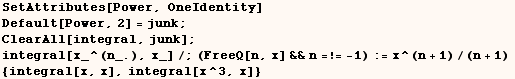Next the value of  Default[Power,2]  is returned to normal, and Power is
protected again.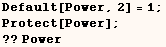Attributes[Power] = {Listable, NumericFunction, OneIdentity, Protected}
 Power/:Default[Power, 2] = 1

There are only three functions in the Mathematica kernel (Version 4.2 or
earlier) that have built-in values for Default[symb, ___].  The functions are
Times, Plus, and Power with the default values ( Default[Times]=1,
Default[Plus]=0,  Default[Power,2]=1 ).

The cells above demonstrated the way OneIdentity and the value  assigned to Default[Power,2] affects pattern matching.  A similar  demonstration is given below on a generic function (f).  In the next cell (f)  has no attributes.  Here 5 doesn't match the pattern f[a_,b_.] and the rule isn't used.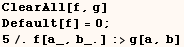Next the same thing is done when (f) has the attribute OneIdentity.  The
OneIdentity attribute permits the pattern matcher to treat 5 as f[5,0].  A
zero was used as the second argument of (f) because the second argument in
the pattern is optional and (f) has a default value of zero.  Once 5 is
treated as f[5,0] the rule can be used.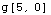In the next cell Default[f] has no assigned value, but 5 still matches the
pattern f[a_,b_:t].   Here again the second argument of (f) is optional.
Since (f) has the OneIdentity attribute the pattern matcher treats 5 as
f[5,t] and the rule is applied.  The rule wouldn't be applied if (f) didn't
have the OneIdentity attribute.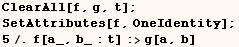OneIdentity and the Flat attribute

OneIdentity also has an effect (independent of optional arguments) for  symbols that have the attributes Flat and OneIdentity.  Recall the effect the Flat attribute has on pattern  matching.  In the line below (f) has the Flat attribute and no other  attributes.  For the given expression (f) has more than two arguments so the  rule can't be applied as entered.  However the pattern matcher knows (f) is  Flat, and it treats f[a1,a2,a3,a4] as the equivalent expression  f[f[a1],f[a2,a3,a4]] in which case the outer (f) has two arguments.  The rule  can then be applied and we are left with {f[a1],f[a2,a3,a4]}.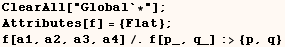In the next example (f) has the attributes Flat and OneIdentity.  The
OneIdentity attribute prevents the pattern matcher from wrapping (f) around a
single argument.  As a result the pattern matcher treats f[a1,a2,a3,a4] as
f[a1,f[a2,a3,a4]] and we are left with {a1,f[a2,a3,a4]} after the rule is
applied.  Notice how this differs from the previous result.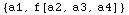In the last example the rule f[p_,q_]:→{p,q} was used with
ReplaceAll (expr/.rule).  If ReplaceRepeated (expr//.rule) is used the rule
is applied as many times as possible. You can try it if you like.

In the next output we see Join has the attributes Flat and OneIdentity, and
the previous demonstration is repeated using Join instead of (f).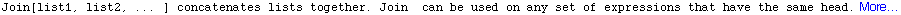Attributes[Join] = {Flat, OneIdentity, Protected}

Since Join has the attributes Flat and OneIdentity the pattern matcher  treats Join[a1,a2,a3,a4] as Join[a1,Join[a2,a3,a4]], and the rule is used.   Here use of HoldPattern is required or Join[a_,b_] would evaluate to Pattern[a,_,b,_] before  pattern matching is finished.In the next cell Join doesn't have the OneIdentity attribute, and the pattern
matcher treats Join[a1,a2,a3,a4] as Join[Join[a1],Join[a2,a3,a4]], and the
rule is used.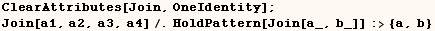Before continuing we should restore the OneIdentity attribute to Join.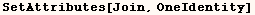In the cells below we look at other examples where a function (f) has the  attributes Flat, OneIdentity. Consider the next cell where (f) still has the  attributes Flat and OneIdentity.  In this case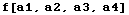evaluates to, and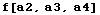evaluates to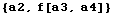.  Then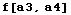evaluates to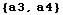.  When evaluation is complete we have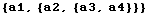.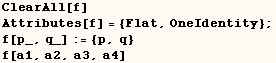The next input is the same as the last input except (f) does not have the  OneIdentity attribute.  In this case the pattern matcher treats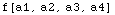aswhich evaluates to.  Then the pattern matcher treatsas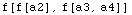which evaluates to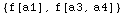.  Then the pattern matcher treatsaswhich evaluates to.  When evaluation is complete we have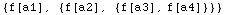. Notice how this differs from the previous result.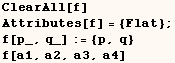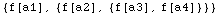In the next cell f[a,b,2,c,d] is treated as f[f[a],f[b,2,c,d]] and other
variations such as f[f[a,b],f[2,c,d]], but none have two arguments under (f)
where the first argument has the head Integer.  The pattern matcher is unable
to find a pattern that matches so the rule is not used.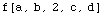Below the very same thing is done except (f) now has the attributes Flat and
OneIdentity.  In this case the pattern matcher treats f[a,b,2,c,d]  as
f[a,b,f[2,f[c,d]]]  in which case f[2,f[c,d]] matches the required pattern.
Then f[2,f[c,d]] evaluates to {2,f[c,d]} and f[c,d] doesn't evaluate further
because the first argument isn't an Integer. We then have f[a,b,{2,f[c,d]}].
The outer (f) doesn't evaluate further because the pattern matcher can't make
it match the required pattern.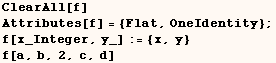For the input below the condition was evaluated for different ways of nesting
layers of (f).  Every possible subexpression matching the pattern was tried
in the search for a subexpression that matches the pattern f[x_,y_] and makes
the condition True.  The condition (a print statement) never evaluated to
True so the rule was not applied.  While searching for a way to make the
condition True, the expression f[a1, a2, a3, a4] was treated as (in this
order):

f[f[a1],f[a2,a3,a4]],

f[f[a1,a2],f[a3,a4]],

f[f[a1,a2,a3],f[a4]],

f[f[f[a1],f[a2,a3]],f[a4]],

f[f[f[a1,a2],f[a3]],f[a4]],

f[f[a1],f[f[a2],f[a3,a4]]],

f[f[f[a1],f[a2]],f[a3,a4]],

f[f[a1],f[f[a2],f[a3]],f[a4]],

f[f[a1,a2],f[f[a3],f[a4]]]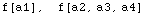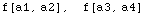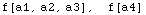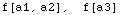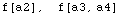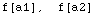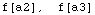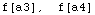Created by Mathematica  (May 16, 2004)